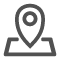# 网络训练时为何要加正则化，有哪些手段?在深度学习中，加入正则化是为了防止过拟合(overfitting)现象的发生。过拟合指的是模型在训练数据上表现得很好，但在未见过的测试数据上表现不佳，因为模型在训练过程中过度拟合了训练数据的噪声和细节。正则化的目的是限制模型的复杂性，以提高其在未知数据上的泛化能力。

常见的正则化技术有：

## 1.L1正则化(L1 regularization)

也称为Lasso正则化，通过增加模型参数的绝对值之和作为惩罚项来限制模型复杂性。它可以促使模型产生稀疏权重，即许多参数变为零，从而实现特征选择的效果。

## 2.L2正则化(L2 regularization)

也称为Ridge正则化，通过增加模型参数的平方和作为惩罚项来限制模型复杂性。与L1正则化不同，L2正则化倾向于让参数接近于零，但不严格等于零。

## 3.Dropout正则化

在训练过程中，随机将一部分神经元的输出置为零，以减少不同神经元之间的依赖性。这样做可以促使网络学习更鲁棒的特征，并减少过拟合。

现在让我们通过Python代码演示如何在神经网络中使用L2正则化。

首先，我们需要导入必要的库和模块：

```import numpy as np
import tensorflow as tf
from tensorflow.keras.models import Sequential
from tensorflow.keras.layers import Dense
from tensorflow.keras.regularizers import l2```

然后，我们准备一些虚拟的训练数据：

```# 生成虚拟的训练数据
np.random.seed(42)
X_train = np.random.random((1000, 10))
y_train = np.random.randint(2, size=(1000, 1))

X_test = np.random.random((200, 10))
y_test = np.random.randint(2, size=(200, 1))```

接下来，我们创建一个包含L2正则化的神经网络模型：

```# 创建神经网络模型
model = Sequential()

# 编译模型

在上面的代码中，我们使用了kernel_regularizer=l2(0.01)来在每一层的权重上添加L2正则化，其中0.01是正则化的系数，控制正则化的强度。较大的正则化系数会对权重施加更强的惩罚，使权重更加接近于零。

最后，我们进行模型的训练并评估：

```# 训练模型
model.fit(X_train, y_train, batch_size=32, epochs=50, validation_data=(X_test, y_test))

# 评估模型在测试集上的性能
loss, accuracy = model.evaluate(X_test, y_test)
print(f'Test accuracy: {accuracy:.4f}')```

通过这样的操作，我们在神经网络中应用了L2正则化，有助于防止过拟合，提高了模型在未知数据上的泛化能力。